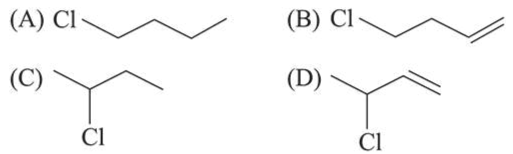# The decreasing order of reactivity towards dehydrohalogenation

Question:

The decreasing order of reactivity towards dehydrohalogenation $\left(E_{1}\right)$ reaction of the following compounds is:1. $\mathrm{D}>\mathrm{B}>\mathrm{C}>\mathrm{A}$

2. $\mathrm{B}>\mathrm{D}>\mathrm{A}>\mathrm{C}$

3. $\mathrm{B}>\mathrm{D}>\mathrm{C}>\mathrm{A}$

4. $B>A>D>C$

Correct Option: 1

Solution:

$\mathrm{E}_{1}$ reaction proceeds via carbocation formation, therefore greater the stability of carbocation, faster will be the $\mathrm{E}_{1}$ reaction.

Thus correct decreasing order of the given halides towards dehydrohalogenation by $E_{1}$ is

$\mathrm{D}>\mathrm{B}>\mathrm{C}>\mathrm{A}$